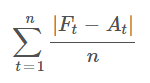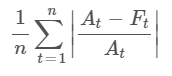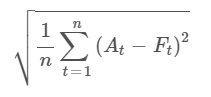# Error Measures

Error measures serve to measure the accuracy of a prediction. It helps both during experimenting and for the tracking of models already deployed in production. TIM returns not only the overall accuracy (all) but also the accuracy across days (bin) and for individual samples ahead (samplesAhead). The number of returned bins, samples ahead and the calculation period (in-sample/out-of-sample) depends on the forecasting scenario and defined in-sample and out-of-sample rows.

The example below gives a general overview of the JSON error measures output. What `Accuracies{}` contains will be the part of the following section as this differs based on the type of task.

## Structure of the output​

``{  "all": {    "name": "all",    "inSample": Accuracies{},    "outOfSample": Accuracies{}  },  "bin": [    {      "name": "S+1:S+24",      "inSample": Accuracies{},      "outOfSample": Accuracies{}    }  ],  "samplesAhead": [    {      "name": "1",      "inSample": Accuracies{},      "outOfSample": Accuracies{}    },    {      "name": "2",      "inSample": Accuracies{},      "outOfSample": Accuracies{}    },    {      "name": "3",      "inSample": Accuracies{},      "outOfSample": Accuracies{}    }  ]}``

## Accuracies​

To reasonably measure the performance of TIM, different performance metrics are used based on the type of task. In forecasting tasks, it is critical to look at the differences between actual and estimated values of the target, whereas in classification, it makes more sense to measure the ability to determine the class of the target correctly.

### Forecasting​

For the forecasting tasks, the accuracy output contains the following performance metrics.

``{      "mae": 2537.635976265567,      "mape": 7.754281689078127,      "rmse": 3817.783568881397}``

#### MAE​

The Mean Absolute Error (MAE) is the most straightforward measure of forecasting accuracy. It is an arithmetic average of the absolute errors between the forecasted and actual values. MAE reveals the expected size of the forecast error on average.#### MAPE​

The Mean Absolute Percentage Error (MAPE) is a strong, commonly used forecasting metric, thanks to its highly intuitive interpretation in terms of relative error. It is the average of the sum of individual absolute percentage errors between the actual and forecasted values. It is not appropriate to use this measure for time series with values close to zero as a slight deviation in error can cause very large changes in the absolute percentage error in these cases.#### RMSE​

The Root Mean Square Error (RMSE) is a frequently used forecasting measure utilized to estimate positional accuracy. It is the square root of the average of the sum of squared differences between the actuals and estimates. RMSE represents the concentration level of the data around the line of best fit.For binary classification tasks, the accuracy output contains the following performance metrics.

``{        "accuracy": 0.9508081067213956,        "AUC": 0.9499988407044577,        "confusionMatrix": {          "truePositive": 12726,          "trueNegative": 2099,          "falsePositive": 426,          "falseNegative": 341}``

#### Accuracy​

The most simple metric - accuracy - represents the ratio of correct predictions to the total amount of predictions made.

#### AUC​

The AUC represents the ranking ability of prediction scores. It denotes the probability that TIM will rank a randomly selected positive class (1) higher than a randomly selected negative class (0).

The section dedicated to AUC explains this metric in more detail.

#### Confusion Matrix​

The confusion matrix summarizes TIM's ability to classify points correctly. Concrete nominal values express this ability for four possible situations - true positives and true negatives (desired outcomes) and false positives and false negatives (wrong outcomes).

The section dedicated to the confusion matrix provides more detail on this topic.